Theory & Practice - Issue 04 - Curved Surfaces, Part II by (07 June 2000) Return to The Archives
 IntroductionLast time, we introduced curved surfaces and some methods to implement them. Now let's take a look at some issues in more detail.

 Some OptimizationThe deCasteljau algorithm isn't the most efficient algorithm for tesselating Bezier surfaces. (See the Links for a Gamasutra article on forward differencing.) However, we can make things more efficient by precalculating some stuff for our surface tesselator.Take, for example, the curved triangles we've been talking about. Each edge of each curved triangle is a 3D Bezier curve (distinct from a 3D Bezier surface). Now, during our tesselation of the triangle we'll be forming new vertices along the Bezier edges between already existing vertices. Without any optimization, we'd have to calculate P(t) = (1-t)3 * P0 + 3t(1-t)2 * P1 + 3t2(1-t) * P2 + t3 * P3 for each new vertex (if you recall, this is equation 1 from last time). Since the coefficients to Pn depend only on t, we can speed things up by computing the coefficients first for a set of possible t's we'll use, thereby forming a small lookup table. Whenever we need to form a new vertex based on two other vertices, we simply call up those coefficients to be multiplied by the four points from the vertices and their vectors.For fractal tesselation, this works wonderfully. The lookup table consists of one entry: the one corresponding to t = 0.5 . This is because we'll always just be getting the midpoints.For linear tesselation, this works less wonderfully. When we're forming the lookup table for an 8x8x8 triangle, we will have store values for 1/8...7/8, 1/7...6/7, and so on until we finally end with 1/3, 2/3, 1/2. Some of these will overlap, so we won't actually have 7+6+5+4+3+2+1 entries. For example, 1/2 and 4/8, are the same number. But there will still be quite a few.The good news is, the table still speeds up calculation, since some values don't have to be recalculated. Plus, if things are done right, the table may well find its way into the secondary cache of the processor. And once it's there, things go even quicker.

 Cracks In The SurfaceWhen you change the level-of-detail between two adjacent patches or primitives, cracks can form between the two edges. This is because a curved edge for one patch or primitive might be approximated using five lines segments, while the same edge for the adjacent patch or primitive might be approximated with two. One way to get around this is to calculate level-of-detail on a per-mesh basis (where a mesh is a complete collection of connected primitives), so the whole mesh has the same LOD. However, this does not always work well. For example, if you have a landscape engine, the entire landscape can be one large mesh. Having one level of detail for an entire landscape is usually not a good idea. You want parts that are closer to be rendered with more detail than things further away.

Instead, we can calculate the level-of-detail per edge. In that case the level of detail for two patches, for the same edge, will be the same. But then, we might have patches where different edges have different levels of detail (and hence a different number of lines approximating different curved edges). How do we tesselate the patch they represent and produce the right level of detail at each edge? It turns out this can be done.

Suppose you have a triangle, where one edge should be approximated with 4 line segments, another with 4 and one with 8. You can split this triangle into two triangles, and both triangles have "4-segment edges".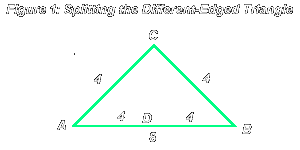In this case, you split the triangle ABC by getting the midpoint of the edge AC. You form a new vertex there, and the normal vector for that vertex is the average of the normals for vertices A and C (as you did before). Anyway, you get two triangles: DBC and BCD. Basically, when you split a triangle along some edge MN obtaining a new vertex O, you get two triangles in which two of the vertices are the same, but in one of the triangles, vertex M is replaced by O and in the other, vertex N is replaced by O.

Here's a general method for taking a triangle whose edges all have different levels of detail, and splitting it into several triangles, each of which has edges all with the same level of detail.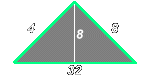Begin by considering the entire original triangle. Take the edge with the largest number of divisions. Create a new vertex on that edge, and divide the original triangle into two. Besides splitting the original edge, you have also created a new edge. Mark that edge with the second-largest number of divisions found in any of the edges, in this case 8.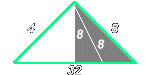Now consider the triangle on the right. That triangle is now 16 by 8 by 8. We do the same: split the edge with 16 divisions, forming two 8 by 8 by 8 triangles.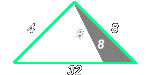Consider the triangle at the extreme right. It is 8 by 8 by 8. There is no need to split it further. We can now tesselate the curved triangle and send it to the rasterizer to be drawn. (3 fractal splits.)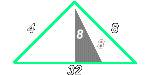Consider the other triangle at the right. It's also 8 by 8 by 8. We tesselate it and draw. (3 fractal splits).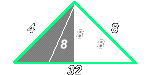Now we do the other side, which is a 4 by 8 by 16 triangle. We split it according to the rules, obtaining one 8x8x8 triangle and one 4x8x8 triangle.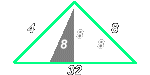Consider the 8x8x8 triangle (on the right side). We don't need to split it further, so we tesselate and draw.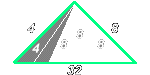Now consider the 4x8x8 triangle (on the left side). We split it according to the rules, obtaining a 4x4x4 triangle and a 4x8x4 triangle.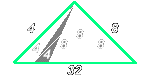Consider the 4x8x4 triangle. We split it according to the rules, obtaining two 4x4x4 triangles.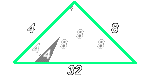We tesselate the bottom 4x4x4 triangle and draw it. (2 fractal splits)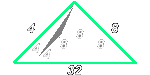Then we do the same to the top one.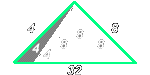Finally, we tesselate the leftmost 4x4x4 triangle and send it to the rasterizer to be drawn.

One point: in the original triangle, each edge should have a number of splits equal to a power of 2. This means that, if you increase or decrease the LOD, the number of splits along an edge must keep being a power of 2, so you can't be VERY gradual with it. On the other hand, this is not a very big restriction, since stuff like increasing detail by 2 each time generally produces good looking results.

 Some IssuesCurved PolygonsIf you want to implement curved polygons with arbitrary vertex counts, you should use the triangle method. Each vertex will have two normal vectors. Then the polygons will be split into triangles, and the triangles will be tesselated as described above. There is only one small problem with this: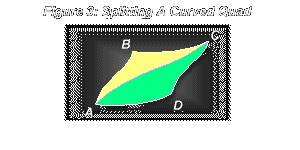The problem is, if you split this quad triangles as shown, changing the vertex D has no effect on the triangle ABC and changing the vertex B has no effect on the triangle ACD. If instead split the quad into triangles ABD and BCD we'd have the same problem. This is not a potentially large problem, but you should keep in mind that what's really being tesselated aren't actual polygons but the triangles that make them up. ClippingThere is also the issue of whether you clip before or after you tesselate a curved surface. My answer is, it depends on what you're doing. If you're doing the clipping and tesselation yourself, then you should clip the original curved polygon (when you clip a vertex remember to adjust all of its attributes: x, y, z, u, v, perhaps the colors and alpha, and don't forget the normal vectors!). Then you can tesselate the polygon. There is one problem that might arise, which deals with the fact that a triangle, when clipped, can become either a triangle or a quad. If you get a quad you'll have to split it into two triangles before actually tesselating it.On the other hand, if you're letting some library or API do the tesselation and clipping for you, then you don't even really need to know the tesselation algorithms in this tutorial. Everything will be handled for you. But it's still a nice read :) Anyway, if you're not the one in charge of the clipping of scenes and the tesselation of curved surfaces, just pass the curved surfaces to the rendering pipeline and let it do its job.If you're doing the tesselation but not the clipping, you don't have much choice but tesselate first, send the vertices, and let the clipping be performed for you. This is the case if you're using an API like OpenGL to clip for you. Who knows, with the new cards coming out like the GeForce II, perhaps this will be handled in hardware much faster, and you can go on with other stuff, processing in parallel on the CPU, and spending more time on things like physics and scripts.

 Data Structures (An Aside)While the interpolation magic allows us to produce these smooth transitions, curved surfaces don't perform miracles in storing the shapes of objects. The more complex your surface, the more patches you'll need, and the more information you'll need to store. A basic principle in understanding information storage is, no matter what format you use to store it, the worst case in that format will be at least as big as the information itself. To represent any 3D point, for example, you will have to have 3 values, whether they are in Cartesian coordinates, spherical or cylindrical coordinates, or any other system you can think up. If you try to represent an arbitrary string of 1s and 0s with another string of 1s and 0s you will have to use a string with at least the same length. The way compression algorithms work is they assume something about the data, or they hope something will happen, such as some symbols repeating more than others. And it is more probable than not, for example, that in a large file many symbols will repeat over and over. So these algorithms do in fact succeed in compressing an arbitrary file -- most of the time. And that's what makes them useful.A similar principle applies in modeling physical processes. Instead of tracking every single molecule in the water and where it goes, for example, we have a model based on fluid dynamics. It all depends on how much precision we want. If we just need to know the overall effect, then given a large stream of molecules, we can expect where they will roughly end up, without ever thinking about the individual molecules. The same approach applies in modeling 3D worlds. In creating games, our goal is not to perfectly recreate every single molecule of each object; we can simply store enough to re-create the scene with enough realism for our audience -- the player. If we don't care about being detailed and precise, then we can get rid of some of the information, and store less. So we can "cheat" somewhat, but as a result we potentially save a lot of processing time and the game runs faster.

 ConclusionSo, we've covered curved surfaces and some relevant issues. Unlike some other articles, this was a tutorial, and I hope that at the end you understood enough of what I was talking about to be able to implement curved surfaces (after a bit of experimentation). What I usually do after I read tutorials on advanced topics is try to understand the basic idea, and once I've gotten a good picture of what goes on, I re-read the tutorial and understand the details. Perhaps that could help you, too... let me know :)So, let's review the benefits of curved surfaces. When you implement curved surfaces, you can specify cool structures with less information. You can also dynamically modify the curved patches or triangles as individual units, affecting the entire curve. Level of detail can be taken into account when tesselating curved surfaces, so you get more detail up close and less as you go further away. Since you have vertex normals, you can use them not only for curved surfaces but also for smooth shading. Finally, I'd like to remark on something interesting which I won't go into, and that is: it is known that in human beings the eye and the brain working together perceive many objects by just noticing the outlines of shapes and the colors at the outlines, and then interpolating smoothly between those colors. (For example, try drawing horizontal strips on a piece of paper, each about .5 inches high, the strip at the top being white, then going through the grays and to black on the bottom. Now look at it and you can see color being interpolated across each strip, although the strip itself is one color.)So apart from the hype, curved surfaces are quite useful and it's usually a good idea to implement them in a modern 3D engine.As usual, drop me a line and tell me what you thought of this issue.

 Links And AcknowledgementsYou can find a tutorial on Bezier curves and surfaces here.Get more information on bicubic Bezier patches and forward differencing in this article.Here's an article by Gabe Kruger on Gamasutra, dealing with curved surfaces and OpenGL.An interesting lighting article entitled "Bounded radiosity on curved surfaces"